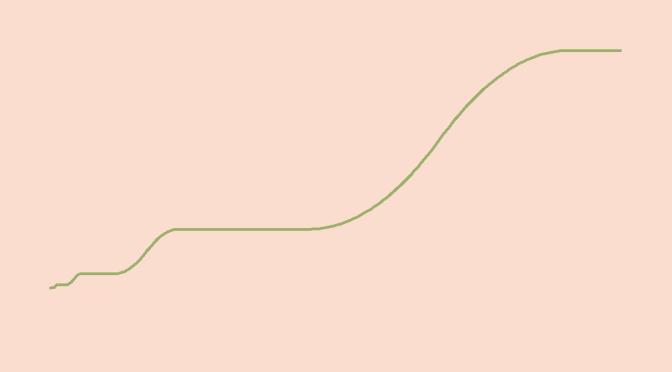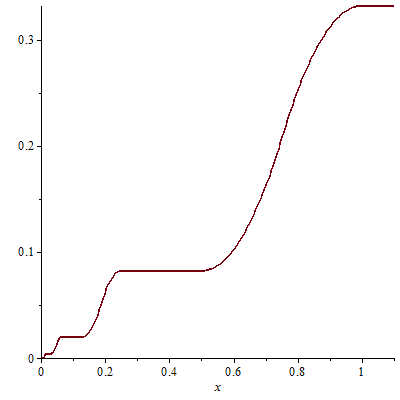# A differentiable function except at one point with a bounded derivative

We build here a continuous function of one real variable whose derivative exists except at $$0$$ and is bounded on $$\mathbb{R^*}$$.

We start with the even and piecewise linear function $$g$$ defined on $$[0,+\infty)$$ with following values:
$g(x)= \left\{ \begin{array}{ll} 0 & \mbox{if } x =0\\ 0 & \mbox{if } x \in \{\frac{k}{4^n};(k,n) \in \{1,2,4\} \times \mathbb{N^*}\}\\ 1 & \mbox{if } x \in \{\frac{3}{4^n};n \in \mathbb{N^*}\}\\ \end{array} \right.$
The picture below gives an idea of the graph of $$g$$ for positive values.$$g$$ is positive, bounded by $$1$$ and continuous on $$\mathbb{R}$$ except at $$0$$. The support of $$g$$ is included in the interval $$[-1,1]$$. Therefore $$g$$ is integrable on every interval of $$\mathbb{R}$$ (both Riemman-integrable and Lebesgue-integrable).

We then define $$\displaystyle f(x)=\int_0^x g(t) dt$$. $$f$$ has following properties:

• $$f$$ is continuous on $$\mathbb{R}$$ as defined as the integral of a function.
• For $$x \in \mathbb{R}^*$$, $$f^\prime(x)=g(x)$$. Which means that $$f$$ is differentiable on $$\mathbb{R}^*$$ with a bounded derivative as $$\vert f^\prime(x)\vert = \vert g(x) \vert \le 1$$ for $$x \in \mathbb{R}^*$$.
• $$f(0)=0$$

$$f$$ graph looks like:Let’s analyze deeper $$f$$ for some real values. For $$n \ge 1$$ integer we have:
$\int^1_{\frac{1}{4^n}} g(t) dt = \sum_{k=1}^n \frac{1}{4^n} = \frac{1}{3}(1-\frac{1}{4^n})$ as this integral is the sum of the areas of triangles having a basis width equal to $$\frac{2}{4^k}$$ and to $$1$$ for their heights. In particular, as $$n \rightarrow +\infty$$ we get $$\displaystyle f(1)=\int_0^1 g(t) dt = \frac{1}{3}$$ and therefore:
$f(\frac{1}{4^n})=\int_0^1 g(t) dt – \int^1_{\frac{1}{4^n}} g(t) dt = \frac{1}{3 \cdot 4^n} = f(\frac{2}{4^n})$ because $$g$$ vanishes on all intervals $$[\frac{1}{4^n},\frac{2}{4^n}]$$.

For all $$n \in \mathbb{N^*}$$ we have:
$\frac{f(\frac{1}{4^n})-f(0)}{\frac{1}{4^n}-0}=\frac{1}{3} \mbox{ and } \frac{f(\frac{2}{4^n})-f(0)}{\frac{2}{4^n}-0}=\frac{1}{6}$As $$\lim\limits_{n \rightarrow +\infty} \frac{1}{4^n} = \lim\limits_{n \rightarrow +\infty} \frac{2}{4^n} = 0$$, we can conclude that $$f$$ is not differentiable at $$0$$.